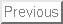Dynamic Programming

Dynamic programming is a very powerful, general tool for solving optimization problems on left-right-ordered items such as character strings. Once understood it is relatively easy to apply, but many people have a difficult time understanding it.

Dynamic programming looks like magic until you have seen enough examples. Start by reviewing the binomial coefficient function in the combinatorics section, as an example of how we stored partial results to help us compute what we were looking for.

Floyd's all-pairs shortest-path algorithm discussed in the graph algorithms chapter is another example of dynamic programming.

Greedy algorithms focus on making the best local choice at each decision point. For example, a natural way to compute a shortest path fromto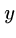might be to walk out of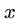, repeatedly following the cheapest edge until we get to. Natural, but wrong! In the absence of a correctness proof such greedy algorithms are very likely to fail.

Dynamic programming gives us a way to design custom algorithms which systematically search all possibilities (thus guaranteeing correctness) while storing results to avoid recomputing (thus providing efficiency).

Evaluating Recurrence Relations

Dynamic programming algorithms are defined by recursive algorithms/functions that describe the solution to the entire problem in terms of solutions to smaller problems. Backtracking is one such recursive procedure we have seen, as is depth-first search in graphs.

Efficiency in any such recursive algorithm requires storing enough information to avoid repeating computations we have done before. Depth-first search in graphs is efficient because we mark the vertices we have visited so we don't visit them again.

Dynamic programming is a technique for efficiently implementing a recursive algorithm by storing partial results. The trick is to see that the naive recursive algorithm repeatedly computes the same subproblems over and over and over again. If so, storing the answers to them in a table instead of recomputing can lead to an efficient algorithm.

Thus we must first hunt for a correct recursive algorithm - later we can worry about speeding it up by using a results matrix.

Edit Distance

Misspellings and changes in word usage (Thou shalt not kill'' morphs into You should not murder.'') make approximate pattern matching an important problem

If we are to deal with inexact string matching, we must first define a cost function telling us how far apart two strings are, i.e., a distance measure between pairs of strings. A reasonable distance measure minimizes the cost of the changes which have to be made to convert one string to another. There are three natural types of changes:

• Substitution - Change a single character from pattern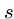to a different character in text, such as changing shot'' to spot''.

• Insertion - Insert a single character into patternto help it match text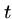, such as changing ago'' to agog''.

• Deletion - Delete a single character from patternto help it match text, such as changing hour'' to our''.

Properly posing the question of string similarity requires us to set the cost of each of these string transform operations. Setting each operation to cost one step defines the edit distance between two strings.

Recursive Algorithm

We can compute the edit distance with recursive algorithm using the observation that the last character in the string must either be matched, substituted, inserted, or deleted. If we knew the cost of editing the three pairs of smaller strings, we could decide which option leads to the best solution and choose that option accordingly. We can learn this cost, through the magic of recursion:


#define MATCH     0       /* enumerated type symbol for match */
#define INSERT    1       /* enumerated type symbol for insert */
#define DELETE    2       /* enumerated type symbol for delete */

int string_compare(char *s, char *t, int i, int j)
{
int k;                  /* counter */
int opt;             /* cost of the three options */
int lowest_cost;        /* lowest cost */

if (i == 0) return(j * indel(' '));
if (j == 0) return(i * indel(' '));

opt[MATCH] = string_compare(s,t,i-1,j-1) + match(s[i],t[j]);
opt[INSERT] = string_compare(s,t,i,j-1) + indel(t[j]);
opt[DELETE] = string_compare(s,t,i-1,j) + indel(s[i]);

lowest_cost = opt[MATCH];
for (k=INSERT; k<=DELETE; k++)
if (opt[k] < lowest_cost) lowest_cost = opt[k];

return( lowest_cost );
}


Speeding it Up

This program is absolutely correct but impossibly slow to compare two 12-character strings! It takes exponential time because it recomputes values again and again and again.

The important observation is that there can only be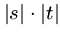possible unique recursive calls, since there are only that many distinct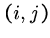pairs to serve as the parameters of recursive calls. By storing the values for each of thesepairs in a table, we can avoid recomputing them and just look them up as needed.

The table is a two-dimensional matrix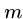where each of thecells contains the cost of the optimal solution of this subproblem, as well as a parent pointer explaining how we got to this location:


typedef struct {
int cost;         /* cost of reaching this cell */
int parent;       /* parent cell */
} cell;

cell m[MAXLEN+1][MAXLEN+1];  /* dynamic programming table */


The dynamic programming version has three differences from the recursive version. First, it gets its intermediate values using table lookup instead of recursive calls. Second, it updates the parent field of each cell, which will enable us to reconstruct the edit-sequence later. Third, it is instrumented using a more general goal_cell() function instead of just returning m[|s|][|t|].cost. This will enable us to apply this routine to a wider class of problems.

Be aware that we adhere to certain unusual string and index conventions in the following routines. In particular, we assume that each string has been padded with an initial blank character, so the first real character of string s sits in s.


int string_compare(char *s, char *t)
{
int i,j,k;              /* counters */
int opt;             /* cost of the three options */

for (i=0; i<MAXLEN; i++) {
row_init(i);
column_init(i);
}

for (i=1; i<strlen(s); i++)
for (j=1; j<strlen(t); j++) {
opt[MATCH] = m[i-1][j-1].cost + match(s[i],t[j]);
opt[INSERT] = m[i][j-1].cost + indel(t[j]);
opt[DELETE] = m[i-1][j].cost + indel(s[i]);

m[i][j].cost = opt[MATCH];
m[i][j].parent = MATCH;
for (k=INSERT; k<=DELETE; k++)
if (opt[k] < m[i][j].cost) {
m[i][j].cost = opt[k];
m[i][j].parent = k;
}
}

goal_cell(s,t,&i,&j);
return( m[i][j].cost );
}


Example

To determine the value of cellwe need three values sitting and waiting for us, namely, the cells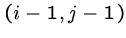,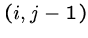, and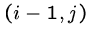. Any evaluation order with this property will do, including the row-major order used in this program.

Below is an example run, showing the cost and parent values turning thou shalt not'' to you should not'' in five moves:


y  o  u  -  s  h  o  u  l  d  -  n  o  t
:   0  1  2  3  4  5  6  7  8  9 10 11 12 13 14
t:   1  1  2  3  4  5  6  7  8  9 10 11 12 13 13
h:   2  2  2  3  4  5  5  6  7  8  9 10 11 12 13
o:   3  3  2  3  4  5  6  5  6  7  8  9 10 11 12
u:   4  4  3  2  3  4  5  6  5  6  7  8  9 10 11
-:   5  5  4  3  2  3  4  5  6  6  7  7  8  9 10
s:   6  6  5  4  3  2  3  4  5  6  7  8  8  9 10
h:   7  7  6  5  4  3  2  3  4  5  6  7  8  9 10
a:   8  8  7  6  5  4  3  3  4  5  6  7  8  9 10
l:   9  9  8  7  6  5  4  4  4  4  5  6  7  8  9
t:  10 10  9  8  7  6  5  5  5  5  5  6  7  8  8
-:  11 11 10  9  8  7  6  6  6  6  6  5  6  7  8
n:  12 12 11 10  9  8  7  7  7  7  7  6  5  6  7
o:  13 13 12 11 10  9  8  7  8  8  8  7  6  5  6
t:  14 14 13 12 11 10  9  8  8  9  9  8  7  6  5


The edit sequence from thou-shalt-not'' to you-should-not'' is DSMMMMMISMSMMMM

Reconstructing the Path

The possible solutions to a given dynamic programming problem are described by paths through the dynamic programming matrix, starting from the initial configuration (the pair of empty strings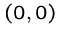) down to the final goal state (the pair of full strings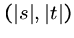).

Reconstructing these decisions is done by walking backward from the goal state, following the parent pointer to an earlier cell. The parent field for m[i,j] tells us whether the transform atwas MATCH, INSERT, or DELETE.

Walking backward reconstructs the solution in reverse order. However, clever use of recursion can do the reversing for us:


reconstruct_path(char *s, char *t, int i, int j)
{
if (m[i][j].parent == -1) return;

if (m[i][j].parent == MATCH) {
reconstruct_path(s,t,i-1,j-1);
match_out(s, t, i, j);
return;
}
if (m[i][j].parent == INSERT) {
reconstruct_path(s,t,i,j-1);
insert_out(t,j);
return;
}
if (m[i][j].parent == DELETE) {
reconstruct_path(s,t,i-1,j);
delete_out(s,i);
return;
}
}


Customizing Edit Distance

• Table Initialization - The functions row_init() and column_init() initialize the zeroth row and column of the dynamic programming table, respectively.

• Penalty Costs - The functions match(c,d) and indel(c) present the costs for transforming character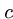to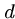and inserting/deleting character. For standard edit distance, match should cost nothing if the characters are identical, and 1 otherwise, while indel returns 1 regardless of what the argument is.

• Goal Cell Identification - The function goal_cell returns the indices of the cell marking the endpoint of the solution. For edit distance, this is defined by the length of the two input strings.

• Traceback Actions - The functions match_out, insert_out, and delete_out perform the appropriate actions for each edit-operation during traceback. For edit distance, this might mean printing out the name of the operation or character involved, as determined by the needs of the application.

Substring Matching
Suppose that we want to find where a short patternbest occurs within a long text, say, searching for Skiena'' in all its misspellings (Skienna, Skena, Skina, ...). Plugging this search into our original edit distance function will achieve little sensitivity, since the vast majority of any edit cost will be that of deleting the body of the text. We want an edit distance search where the cost of starting the match is independent of the position in the text, so that a match in the middle is not prejudiced against. Likewise, the goal state is not necessarily at the end of both strings, but the cheapest place to match the entire pattern somewhere in the text. Modifying these two functions gives us the correct solution:


row_init(int i)
{
m[i].cost = 0;       /* note change */
m[i].parent = -1;    /* note change */
}

goal_cell(char *s, char *t, int *i, int *j)
{
int k;                  /* counter */

*i = strlen(s) - 1;
*j = 0;
for (k=1; k<strlen(t); k++)
if (m[*i][k].cost < m[*i][*j].cost) *j = k;
}


Longest Common Subsequence
Often we are interested in finding the longest scattered string of characters which is included within both words. The longest common subsequence (LCS) between democrat'' and republican" is eca.

A common subsequence is defined by all the identical-character matches in an edit trace. To maximize the number of such traces, we must prevent substitution of non-identical characters. This is done by changing the match-cost function:


int match(char c, char d)
{
if (c == d) return(0);
else return(MAXLEN);
}


Maximum Monotone Subsequence
A numerical sequence is monotonically increasing if the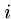th element is at least as big as thest element. The maximum monotone subsequence problem seeks to delete the fewest number of elements from an input stringto leave a monotonically increasing subsequence. Thus a longest increasing subsequence of 243517698'' is 23568.''

In fact, this is just a longest common subsequence problem, where the second string is the elements of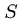sorted in increasing order. Any common sequence of these two must (a) represent characters in proper order in, and (b) use only characters with increasing position in the collating sequence, so the longest one does the job. Of course, this approach can be modified to give the longest decreasing sequence by simply reversing the sorted order.

As you can see, our simple edit distance routine can be made to do many amazing things easily. The trick is observing that your problem is just a special case of approximate string matching.

Assigned Problems

111101 (Is Bigger Smarter?) - Find the longest sequence of elephants whose weights are increasing but whose IQ's are decreasing. Can this be done as a special case of edit distance?

111103 (Weights and Measures) - Find the tallest possible stack of turtles you can build, where each turtle has a strength and weight. Can this be done as a special case of edit distance?

111104 (Unidirectional TSP) - Find the cheapest left-right path across a matrix. Is this shortest path or dynamic programming, or is Dijkstra's algorithm really dynamic programming?

111106 (Ferry Loading) - How can we fit the most amount of cars on a two-lane ferry? Does always putting the next car on the side with the most remaining room solve the problem? Can we exploit the fact that the sum of accumulated car lengths on each lane of the ferry is always an integer?